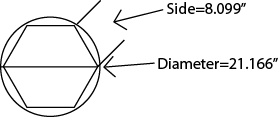First Line Sales
PO Box 200
Vernon, VT 05354

www.firstlinesales.com

Construction Estimating Formulas & Useful Information

Formulas, equations, tables, facts, specifications and other
information useful in planning and estimating construction,
decorating and other projects.

The circumference of a circle can be divided into equal parts with a calculator.  This will allow you to make proportioned shapes within the circle.  For example -stars, triangles, octagons, etc.

To find the divisions, multiply the diameter of the circle by the following multipliers.

 Division Multiplier Division Multiplier 3 .86603 8 (Octagon) .38268 4 .70711 9 .34202 5 .58779 10 .30902 6 .50000 11 .28173 7 .43388 12 .25882

For example:

21.166" x .38268 (from above table) = 8.099"6.  Board Feet:  A board foot is essentially a measure of wood, figured on the called size, not the actual finished size.  Therefore a 2" x 4" x 8" contains 5.33 bd. ft.  The theory is that you pay for what the mill started with before the wood is dried and planed.

Thickness" x Width" x Length in feet, divided by 12 = board feet. T" x W" x L" ÷ 12 = Board ft.

Thickness" x Width" x Length in inches divided by 144 = bd. ft. (T" x W" x L") ÷ 144 = Board ft.

This information is believed to be accurate.  The Polymer Roof Factory or First Line Sales will not be liable for errors and omissions.  Specifications, detail, and other information should be verified by the user.